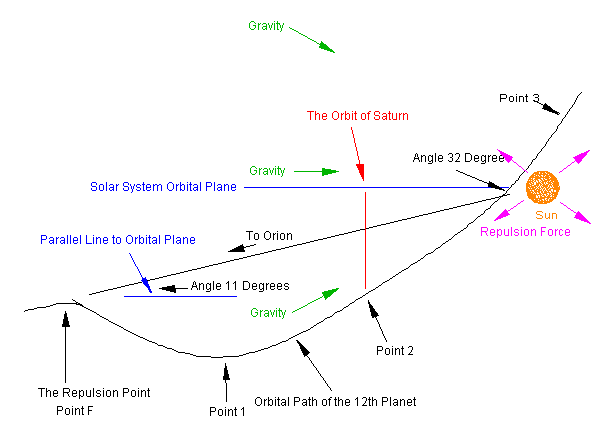The Forces Affecting the 12th Planet Between Points F and AThe Repulsion  Point: It is the point where the repulsion force, still unknown to science pushes the 12th planet away from the solar system orbital plane along the Y & Z-axis.

Point 1: This is the point where the 12th planet reaches the maximum distance away from the solar system orbital plane due to the repulsion force & gravity cancel about the Y and Z axis and force along the X axis is in favor of gravity as the 12th planet turns inward towards after passing this point on a direct path to the Sun.

Point 2: Here the 12th planet reaches its maximum piercing angle to the solar system orbital plane at the orbit of Saturn as the net forces of gravity and repulsion slow forward progression, but maintain a direct line towards the Sun.

Point 3: As the 12th planet leaves the solar system it forms a 32-degree angle between the X & Y-axis with the X-axis as the base. The repulsion force provides outward velocity along the imaginary plane of Z, thus the curved orbit.